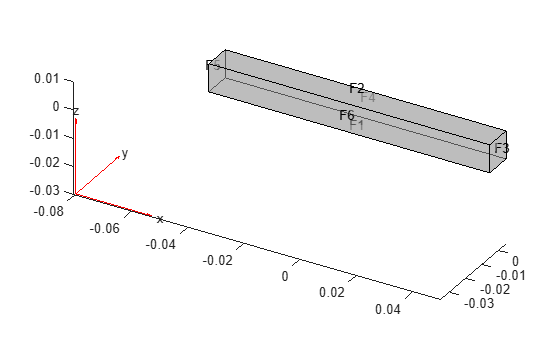ReducedStructuralModel

Reduced order structural model results

Description

A ReducedStructuralModel object contains the stiffness matrix K, mass matrix M, mesh, multipoint constraint reference locations, and IDs of retained degrees of freedom.

To expand this data to a full solution that includes displacement, velocity, and acceleration, use reconstructSolution.

Creation

Reduce a structural model by using the reduce function. This function returns structural results obtained using the Craig-Bampton reduced order method as a ReducedStructuralModel object.

Properties

expand all

Reduced stiffness matrix, returned as a real N-by-N matrix.

• For models without multipoint constraints, N is the sum of the number of retained degrees of freedom and the number of fixed interface modes.

• For models with Nmp multipoint constraints, N is the sum of 6*Nmp and the number of fixed interface modes.

Data Types: double

Reduced mass matrix, returned as a real N-by-N matrix.

• For models without multipoint constraints, N is the sum of the number of retained degrees of freedom and the number of fixed interface modes.

• For models with Nmp multipoint constraints, N is the sum of 6*Nmp and the number of fixed interface modes.

Data Types: double

Number of fixed interface modes, returned as an integer.

Data Types: double

IDs of retained degrees of freedom, returned as a real vector.

Data Types: double

Multipoint constraint reference locations, returned as a real 2-by-Nmp or 3-by-Nmp matrix for a 2-D or 3-D geometry, respectively. Here, Nmp is the number of multipoint constraints. If there are no multipoint constraints, ReferenceLocations is an empty matrix.

Data Types: double

Finite element mesh, returned as a FEMesh object. For details, see FEMesh Properties.

Object Functions

 reconstructSolution Recover full-model transient solution from reduced-order model results

Examples

collapse all

Reduce the model to the fixed interface modes in the specified frequency range and the boundary interface degrees of freedom.

Create a transient structural model for a 3-D problem.

structuralmodel = createpde('structural','transient-solid');

Create a geometry and include it in the model. Plot the geometry.

gm = multicuboid(0.1,0.01,0.01);
structuralmodel.Geometry = gm;
pdegplot(structuralmodel,'FaceLabels','on','FaceAlpha',0.5)Specify the Young's modulus, Poisson's ratio, and mass density of the material.

structuralProperties(structuralmodel,'YoungsModulus',70E9, ...
'PoissonsRatio',0.3, ...
'MassDensity',2700);

Generate a mesh.

generateMesh(structuralmodel);

Specify the ends of the beam as structural superelement interfaces. The reduced-order model technique retains the degrees of freedom on the superelement interfaces while condensing the degrees of freedom on all other boundaries. For better performance, use the set of edges that bound each side of the beam instead of using the entire face.

structuralSEInterface(structuralmodel,'Edge',[4,6,9,10]);
structuralSEInterface(structuralmodel,'Edge',[2,8,11,12]);

Reduce the model to the fixed interface modes in the frequency range [-Inf,500000] and the boundary interface degrees of freedom.

R = reduce(structuralmodel,'FrequencyRange',[-Inf,500000])
R =
ReducedStructuralModel with properties:

K: [166x166 double]
M: [166x166 double]
NumModes: 22
RetainedDoF: [144x1 double]
ReferenceLocations: []
Mesh: [1x1 FEMesh]

expand all